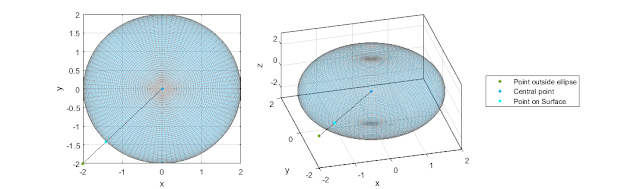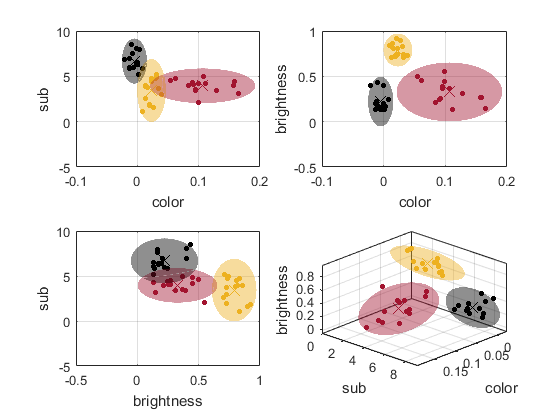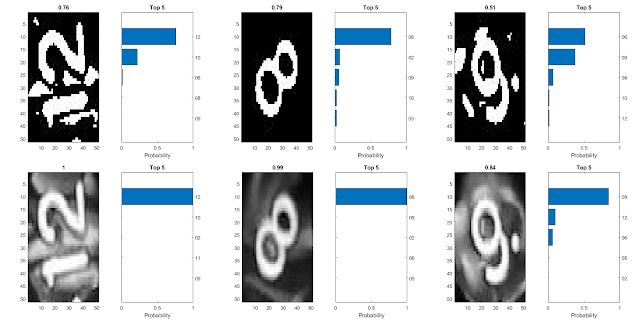## Posts

### Short trip to Krakow (insider hints)Our 2 day Krakow trip

(German) We spontaneously decided to go to Krakow because the Ryanair flight was 24€ back and forth. Because we did not know what to do in Krakau we searched the Internet for all special things we have to consider and what is most important to see.

Money: We have heard a lot of times before that Krakow is super cheap but it is also very European so if you go to the touristic places you still pay a lot for meals and so on.
Exchange: We have taken for the 2 days 200 zloten (polnish Dollar = 58€) at the Airport but it would have been cheaper if we would have done this in Frankfurt.
Transfer: The Transfer from the Airport to the oldtown is quiet cheap (3.8 zloten ~ 1€) and you can buy it also in the busses if you just catch the bus. You don't need any transfers in Krakow because everything is very close there so enjoy the walks along the city. Hostel: Hostels are very cheap! We took the Salve Hostel next to the old town which was 60€ for 2 persons and 2 nights with …

### Matlab: Point on ellipsoidal surface(German) This post is focussing on the solution of calculating a point of an ellipsoidal surface in dependency of to angles like in spherical coordinates.
Mode of operation: This function calculates the closest point on an ellipsoidal surface from an point outside or inside the body in respect to its center. It needs the mesh data of the ellipsoidal body X, Y, Z and the point, where the point with direct connection to the center should be found on the surface. The output parameters are [point, phi, the] = PointOnSurfaceAnalytic(X, Y, Z, x, y, z); the surface point and the angles phi and theta of the ellipsoidal equations. It can be downloaded here.

To derive the formula we start with the ellipsoidal equation:
xa+yb+zc2=1
If we consider 𝝋 to be between the x and y axis and
𝝑 be between the y and z axis it leads to:
y=x tan 𝝋
z=y tan 𝝑
Substitute this in the ellipsoidal equation one gets: ya tan 𝝋+yb+y tan 𝝑c2=1
solve this for y gives:
y=11a tan 𝝋+1b+tan 𝝑c2
so one can calculate x, …

### Matlab: Classification(German) This post describes a Matlab function which classifies images dependent on shape color and so on to differentiate between images like bananas and apples.
Mode of operation: In this function different attributes of images are calculated in values to classify images and seperate them in 2D or 3D. Classification methodes are 'color', 'color2' and 'colorhex' for different color classifications and 'brightness' for the brightness value 'shape', 'std', 'mean', 'cov' and 'sub' are different shape conditions. This function can be downloaded on MathWorks. Following figure shows the classification of apples, bananas and black berries.

It is seen in three dimensions it is clearly classified. Most of the mathematical algortihm are based on magnitude gradient maps to whether calculate shape or substructures. Following figure shows the different input images and their gradient map. In source code give below there is…

### Matlab: Convolutional Network for Dice identification(German) This post describes a Matlab function which uses a 7 layer convolutional network for dice number identification (numbers 1-12). It calls for the earlier proposed image processing methode shown in this post.
Mode of operation: Two methodes were tested. The dice as shown in previous post is just a tiny part of the whole image. With image processing, the dice itself is excluded and saved in a new folder sorted by number. They are loaded in "digitData". The it is splitted in trainigs set (4/5) and test set (1/5 of alle images). All in all I have 996 images and I tested the not processed images and the binare tresholded images, which suprisingly showed a higher accuracy for the non processed images. An all in all accuracy of 99.6% in the training set and 99.2% in the test set was achieved around 5% higher than for the binare images. In this image the value of accuracy is seen what the convolutional net predicts. A good improvement is seen in non processed images compar…

### Matlab: Sorting Songs/Files

(German) This post describes a Matlab function which load out of a folder all songs, process them to a standarized name and save them in a folder in the different interpret folders.
Mode of operation: First of all the path is chosen where the songs are, which should be sorted and then the folder is chosen, where the songs are copied to. Because in many folders are files like "..", ".", "desktop.ini", these are filtered. So now are all names of the file loaded. Next the name (interpret and songname) is devided and also further informations in the name like "(Official Video)" or "[pleer.net]" are cutted out.  Now the first song is taken and if there is a folder existing in the folder where it should be saved, then the song is saved, if it isn't already in the folder. If the interpret folder is not existing right now a new folder is generated with the name of the interpret and the song is copied into it. This is done for all songs o…

### Matlab: Image background removing and cutting(German) This post is about a Matlab function where one can load an images and remove the background, by saving it as a transparent image. The background can consist out of different and blurring colors! Useful for prensentations that the image fits to the slice background.
Mode of operation: This is a GUI based function, so that one can load via interface an images of any type. Now one can use the buttom "Choose BG" to select the background color in an additional appearing image. If it is chosen one see in figure 2 the extracted regions in white and in the lower left corner which color was selected. If one uses now the slider one can increase the range of the color. If the value is 10 and the chosen background color is [50 5 200] then the algorithm is also selecting every pixel with the colors between [40 0 190] and [60 15 210]. This is also seen in the lower left corner.  The buttom "next BG" can be used to select a second additional background. Th…

### Matlab: Image Processing: twelve-sided dice with numbers(German) This post is like the post before and describes the identification of the numbers on a twelve-sided dice with numbers from 1 to 12. Also automatically rolled by the dice rolling machine of  my brother.
Dice identification: First of all the images are loaded into Matlab and the size is reduces to 200x200 pixel size. The with rgb2gray the image is reduces to a gray-scaled image and the gradient is used.
The gradient and the normal image is converted into a binare image with a treshold. Then the gradient images is morphologically closed and multiplied with the normal tresholded image and afterwards morphologically dilated.
After this the big for loop over all images is  executed. The the watershed is applied and the middle of the image with my own function is determined, which just calculate the mean of all columns and rows where the image is one. From this middle a big area is chosen that the rolled number is for sure in this area.
Then the biggest area in the wat…